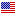4th Edition

# A First Course in Fuzzy Logic

ISBN 9781032475943
458 Pages 36 B/W Illustrations
Published January 21, 2023 by CRC PressFree Shipping (6-12 Business Days)
shipping options

USD \$54.95
ISBN 9781138585089
458 Pages 36 B/W Illustrations
Published November 28, 2018 by Chapman & HallFree Shipping (6-12 Business Days)
shipping options

USD \$150.00
ISBN 9780429505546
458 Pages 36 B/W Illustrations
Published December 7, 2018 by Chapman & Hall

Also available as eBook on:

A First Course in Fuzzy Logic, Fourth Edition is an expanded version of the successful third edition. It provides a comprehensive introduction to the theory and applications of fuzzy logic.

This popular text offers a firm mathematical basis for the calculus of fuzzy concepts necessary for designing intelligent systems and a solid background for readers to pursue further studies and real-world applications.

New in the Fourth Edition:

• Features new results on fuzzy sets of type-2

• Includes quantum probability for uncertainty modeling in social sciences, especially in economics

With its comprehensive updates, this new edition presents all the background necessary for students, instructors and professionals to begin using fuzzy logic in its many—applications in computer science, mathematics, statistics, and engineering.

Hung T. Nguyen is a Professor Emeritus at the Department of Mathematical Sciences, New Mexico State University. He is also an Adjunct Professor of Economics at Chiang Mai University, Thailand.

Carol L. Walker is also a Professor Emeritus at the Department of Mathematical Sciences, New Mexico State University.

Elbert A. Walker is a Professor Emeritus, Department of Mathematical Sciences, New Mexico State University.

The Concept of Fuzziness

Examples. Mathematical modeling. Some operations on fuzzy sets. Fuzziness as uncertainty.

Some Algebra of Fuzzy Sets

Boolean algebras and lattices. Equivalence relations and partitions. Composing mappings. Isomorphisms and homomorphisms. Alpha-cuts. Images of alpha-level sets.

Fuzzy Quantities

Fuzzy quantities. Fuzzy numbers. Fuzzy intervals.

Logical Aspects of Fuzzy Sets

Classical two-valued logic. A three-valued logic. Fuzzy logic. Fuzzy and Lukasiewicz logics. Interval-valued fuzzy logic.

Basic Connectives

t-norms. Generators of t-norms. Isomorphisms of t-norms. Negations. Nilpotent t-norms and negations. T-conforms. De Morgan systems. Groups and t-norms. Interval-valued fuzzy sets. Type-2 fuzzy sets.

Fuzzy implications. Averaging operators. Powers of t-norms. Sensitivity of connectives. Copulas and t-norms.

Fuzzy Relations

Definitions and examples. Binary fuzzy relations. Operations on fuzzy relations. Fuzzy partitions. Fuzzy relations as Chu spaces. Approximate reasoning. Approximate reasoning in expert systems. A simple form of generalized modus ponens. The compositional rule of inference.

Universal Approximation

Fuzzy rule bases. Design methodologies. Some mathematical background. Approximation capability.

Possibility Theory

Probability and uncertainty. Random sets. Possibility measures.

Partial Knowledge

Motivations. Belief functions and incidence algebras. Monotonicity. Beliefs, densities, and allocations. Belief functions on infinite sets. Mobius transforms of set-functions. Reasoning with belief functions. Decision making using belief functions. Rough sets. Conditional events.

Fuzzy Measures

Motivation and definitions. Fuzzy measures and lower probabilities. Fuzzy measures in other areas. Conditional fuzzy measures.

The Choquet Integral

The Lebesgue integral. The Sugeno integral. The Choquet integral.

Fuzzy Modeling and Control

Motivation for fuzzy control. The methodology of fuzzy control. Optimal fuzzy control. An analysis of fuzzy control techniques.

### Biography

Hung T. Nguyen is a Professor Emeritus at the Department of Mathematical Sciences, New Mexico State University. He is also an Adjunct Professor of Economics at Chiang Mai University, Thailand.

Carol L. Walker is also a Professor Emeritus at the Department of Mathematical Sciences, New Mexico State University.

Elbert A. Walker is a Professor Emeritus, Department of Mathematical Sciences, New Mexico State University.

We offer free standard shipping on every order across the globe.